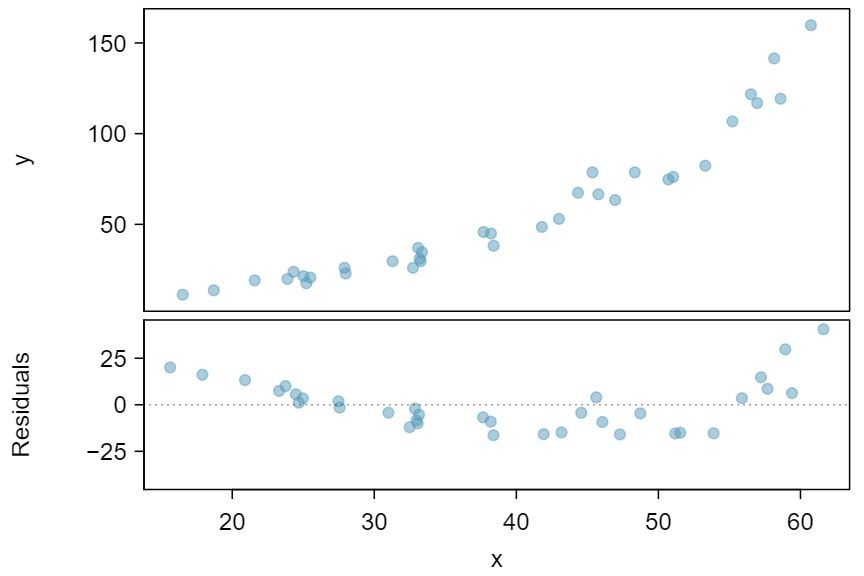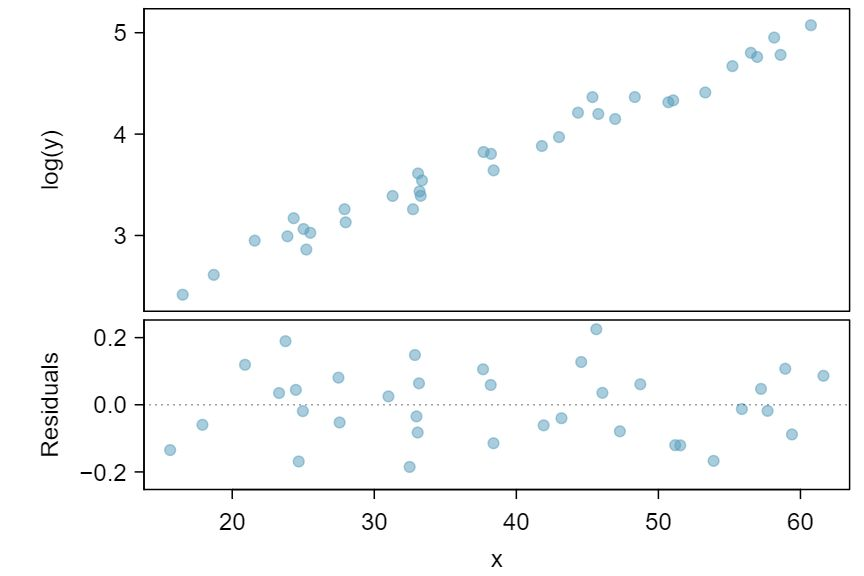## Section8.5Transformations for nonlinear data

### Subsection8.5.1Untransformed

Consider the scatterplot and residual plot in Figure 8.5.2. The regression output is also provided. Would a linear model be a good model for the data shown?

Solution

First, we can note the $R^2$ value is fairly large. However, this alone does not mean that the model is good. Another model might be much better. When assessing the appropriateness of a linear model, we should look at the residual plot. The U pattern in the residual plot tells us the original data is curved. If we inspect the two plots, we can see that for small and large values of $x$ we systematically underestimate $y\text{,}$ whereas for middle values of $x\text{,}$ we systematically overestimate $y\text{.}$ Because of this, the model is not appropriate, and we should not carry out a linear regression $t$-test because the conditions for inference are not met. However, we might be able to use a transformation to linearize the data.The regression equation is:

y = -52.3564 + 2.7842 x

Predictor       Coef   SE Coef         T           P

Constant    -52.3564    7.2757    -7.196       3e-08
x             2.7842    0.1768    15.752     < 2e-16

S = 13.76   R-Sq = 88.26%    R-Sq(adj) = 87.91%


### Subsection8.5.2Transformed

Regression analysis is easier to perform on linear data. When data are nonlinear, we sometimes transform the data in a way that makes the resulting relationship linear. The most common transformation is log (or ln) of the $y$ values. Sometimes we also apply a transformation to the $x$ values. We generally use the residuals as a way to evaluate whether the transformed data are more linear. If so, we can say that a better model has been found.

Using the regression output for the transformed data, write the new linear regression equation

Solution

$\widehat{log(y)} = 1.723 +0.053 x$The regression equation is:

log(y) = 1.722540 + 0.052985 x

Predictor         Coef     SE Coef        T          P

Constant      1.722540    0.056731    30.36    < 2e-16

x             0.052985    0.001378    38.45    < 2e-16

S = 0.1073   R-Sq = 97.82%    R-Sq(adj) = 97.75%


Which of the following statements are true? There may be more than one. 1 Part (a) is false since there is a nonlinear (curved) trend in the data. Part (b) is true. Since the transformed data shows a stronger linear trend, it is a better fit, i.e. Part (c) is false, and Part (d) is true.

1. There is an apparent linear relationship between $x$ and $y\text{.}$

2. There is an apparent linear relationship between $x$ and $\widehat{log(y)}\text{.}$

3. The model provided by Regression I ($\hat{y} = -52.3564 + 2.7842 x$) yields a better fit.

4. The model provided by Regression II ($\widehat{log(y)} = 1.723 +0.053 x$) yields a better fit.